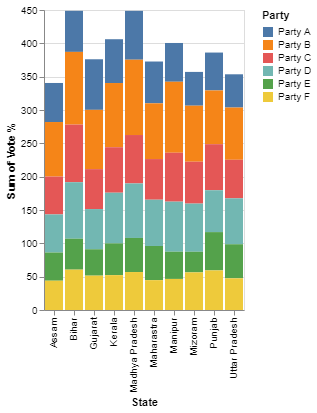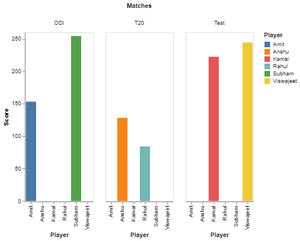# Stacked Bar Chart With Selection Using Altair in Python

• Last Updated : 10 Jun, 2022

Prerequisite: Altair

In this article, We will see Stacked Bar Chart With Selection with Altair python. It is a declarative and interactive visualization tool for Python, based on Visualization Grammar (VeGa), It is simple, user-friendly, and moreover provides clearly interpretable charts. It supports a wide variety of charts like bar, line, scatter, box, violin, and other charts in an interactive way.  Let’s discuss how to create a stacked bar chart with a selection to highlight a particular stack of the bar chart.

### Installation

The following command can be used to install Altair and Pandas like any other python library:

```pip install altair
pip install pandas
pip install altair_viewer```

### Functions Used

• The altair.selection_single() specify the type of selection inside the select method. The method takes parameters that are discussed below.

Syntax: altair.selection_single(type, encodings)

• type – string. Determines the default event processing and data query for the selection. Three types single, multi (shift+ click), interval (drag).
• encodings – List of encoding channels. The corresponding data field values must match for a data tuple to fall within the selection.
• The encode() method is used to map columns to visual attributes of the plot. This method takes in parameters of the x and y-axis and other chart attributes. The mark property is what specifies how exactly those attributes should be represented on the plot.

Syntax: alt.Chart(data).mark_bar().encode(x, y, color)

• x –  The x-axis value
• y – The y-axis value
• color – The color of the mark

### Implementation

Import the Altair and pandas package. Read the dataset using pandas and specify the type of selection we have used the selection_single() method. Then, pass the dataset to the chart() function and make use of the mark_bar().encode() method to pass the x and y-axis values and append the selection object after passing the parameters to the mark_bar() function.

Example 1:

The dataset used for demonstration purposes can be downloaded from here.

## Python3

 `import` `altair as alt ` `import` `pandas as pd ` ` `  `# load the data ` `source ``=` `pd.read_csv(``"test.csv"``) ` ` `  `# specify the type of selection, here single selection is used ` `selector ``=` `alt.selection_single(encodings``=``[``'x'``, ``'color'``]) ` ` `  `# use mark_bar function to plot a stacked bar and specify x and y axis ` `chart ``=` `alt.Chart(source).mark_bar().encode( ` `    ``x``=``'State'``, ` `    ``y``=``'sum(Vote %)'``, ` `    ``color``=``alt.condition(selector, ``'Party'``, alt.value(``'lightgray'``)) ` `).add_selection( ` `    ``selector ` `) ` `# initializer altair_viewer to display the interactive chart ` `alt.renderers.enable(``'altair_viewer'``) ` `chart.show()`

Output:Stacked bar chart

To see the interactive visualization, run the code and it will automatically redirect you to an interactive webpage.

Example 2:

The pandas DataFrame is used for demonstration purposes.

## Python3

 `import` `altair as alt ` `import` `pandas as pd ` ` `  `# creating a custom dataframe ` `data ``=` `pd.DataFrame([[``254``, ``'Subham'``, ``'ODI'``], ` `                    ``[``153``, ``'Amit'``, ``'ODI'``], ` `                    ``[``128``, ``'Anshu'``, ``'T20'``], ` `                    ``[``84``, ``'Rahul'``, ``'T20'``], ` `                    ``[``222``, ``'Kamal'``,``'Test'``], ` `                    ``[``244``, ``'Viswajeet'``,``'Test'``]], ` `                    ``columns``=``[``'Score'``, ``'Player'``, ``'Matches'``]) ` ` `  `# use mark_bar function to plot a stacked bar and specify x and y axis ` `gp_chart ``=` `alt.Chart(data).mark_bar().encode( ` `alt.Column(``'Matches'``),  ` `alt.X(``'Player'``), ` `alt.Y(``'Score'``, axis``=``alt.Axis(grid``=``False``)), ` `alt.Color(``'Player'``)) ` ` `  `# initializer altair_viewer to display the interactive chart ` `alt.renderers.enable(``'altair_viewer'``) ` `gp_chart.display()`

Output:Stacked bar chart

My Personal Notes arrow_drop_up
Recommended Articles
Page :